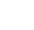Your current browser version is too low, in order to bring you a better browsing effect, please use a higher version browser

You Are Here: home-Blog

# Medium Frequency Induction Furnace Power Calculation

Medium frequency induction furnaces are widely used in various industries for the melting, heating, and heat treatment of metals. The power calculation for these furnaces is crucial in ensuring optimal performance and efficiency. This article will discuss the process of calculating the power required for a medium frequency induction furnace, as well as the factors that influence power selection.

Power Calculation Method:
The power calculation for a medium frequency induction furnace involves several parameters, including the material to be melted, desired melting rate, and furnace efficiency. The formula commonly used for power calculation is as follows:

Power (kW) = (Material mass (kg) × Specific heat (J/kg°C) × Temperature rise (°C)) / Melting time (s) × Furnace efficiency

Material Mass:
The mass of the material to be melted is a crucial factor in determining the power required. It is determined by the desired production rate and the weight of each individual component.

Specific Heat:
Specific heat refers to the amount of heat energy required to raise the temperature of a material by one degree Celsius. Different materials have different specific heat values, and this parameter must be known for accurate power calculation.

Temperature Rise:
The temperature rise represents the desired increase in temperature for the material being melted. It is determined by the melting point of the material and the desired melting temperature.

Melting Time:
The melting time is the duration required to achieve the desired melting of the material. It depends on the melting rate and the mass of the material.

Furnace Efficiency:
Furnace efficiency is an important factor in power calculation as it determines the actual power required to achieve the desired results. It takes into account various losses, such as heat losses through the furnace lining, radiation losses, and electrical losses.

Factors Influencing Power Selection:
Several factors influence the selection of power for a medium frequency induction furnace. These include:

1. Material Type: Different materials have different heat transfer characteristics and melting points, which affect the power requirement.

2. Melting Rate: The desired melting rate determines the power required to achieve the desired production rate.

3. Furnace Size: The size of the furnace determines the volume of material that can be melted at a time, thus impacting the power calculation.

4. Power Supply: The availability and capacity of the power supply influence the selection of the furnace power.

Conclusion:
Calculating the power required for a medium frequency induction furnace is essential for efficient and effective melting and heat treatment processes. By considering factors such as material mass, specific heat, temperature rise, melting time, and furnace efficiency, one can accurately determine the power needed for optimal furnace performance. Additionally, factors like material type, melting rate, furnace size, and power supply capacity should be taken into account when selecting the appropriate power for a medium frequency induction furnace.